# Objective 6. Copy Formulas

Excel provides a quick method to create formulas without typing them and without clicking a toolbar button. This method is known as copying formulas. When a formula is copied from one cell to another, Excel adjusts the cell references to fit the new location of the formula.

Activity 2.9. Copying a Formula with Relative Cell References Using the Fill Handle

1.

In cell E3, type =b3*d3 and press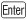. Compare your worksheet with Figure 2.25.

Figure 2.25.

(This item is displayed on page 721 in the print version)The Total Retail Value of all the Polo Shirts in the shop1125equals the Quantity (25) times the Retail Price (selling price) of 45 dollars. In Excel, the asterisk (*) represents multiplication.

2.

Take a moment to study the symbols you will use to perform mathematical operations in Excel, as shown in the table in Figure 2.26.

Figure 2.26. Mathematical Symbols Used in Excel

Operator Symbol

Operation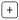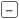Subtraction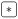Multiplication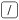Division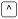Exponentiation

3.

Click cell E3.

You can see that in cells E4:E8, you need a formula similar to the one in E3, but one that refers to the cells in row 4, row 5, and so forth.

4.

With cell E3 selected, position your pointer over the fill handle in the lower right corner of the cell until thepointer displays. Then, drag downward through cell E8. Compare your screen with Figure 2.27.

Figure 2.27.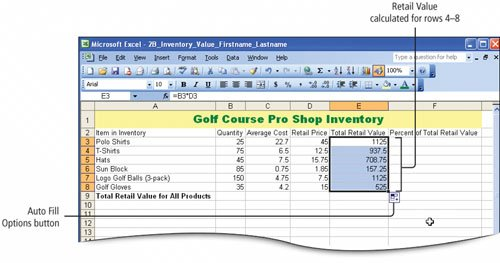5.

Click cell E4, look at the Formula Bar, and notice the formula =B4*D4. Click cell E5, look at the Formula Bar, and notice the formula =B5*D5.

In each row, Excel copied the formula but adjusted the cell references relative to the row number. This is called a relative cell reference. The calculation is the same, but it is performed on the cells in that particular row. Use this quick method to insert numerous formulas into large spreadsheets.

6.

Select the range C3:E3 and click the Currency Style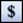button to apply the Accounting format. Select the range C4:E8 and click the

Comma Style button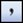. Click cell E9, click the AutoSum button, and then press.

The Accounting format is automatically applied to cell E9. Recall that the Currency Style button applies the Accounting format with dollar signs at the left edge of the cell, and space on the right for a right parenthesis in case of negative numbers. The Comma Style button applies the same format, but without the dollar sign. The Total Retail Value of the six products in inventory is \$4,578.50. The format of the cell containing the formula is the same as the format of the cells used in the formula. If the formula uses a range of cells and those cells contain a mix of formats, the format from the cell in the upper left corner of the range is applied.

7.

Save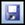your workbook.

Another Way: To Copy Formulas

You can copy formulas with relative cell references using the Copy and Paste commands. Click the cell containing the first formula, and then click the Copy button. Select the range to which you want to copy formulas, and then click the Paste button. The formula will be copied and the relative cell references adjusted accordingly. The Copy and Paste commands can also be initiated from the Edit menu or with the keyboard shortcuts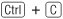and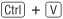, respectively.

Activity 2.10. Copying Formulas Containing Absolute Cell References

You have seen that a relative cell reference refers to cells by their position in relation to the cell that contains the formula. Absolute cell references, on the other hand, refer to cells by their fixed position in the worksheet, for example, the total in cell E9.

A relative cell reference automatically adjusts when a formula is copied. An absolute cell reference does not adjust; rather, it remains the same when the formula is copiedand there are times when you will want to do this.

 1. Click cell F3, type = and then click cell E3. Type / and then click cell E9. The formula created, =E3/E9, indicates that the value in cell E3 will be divided by the value in cell E9. Why? Because Mr. Peterson wants to know the percentage by which each product's Total Retail Value makes up the Total Retail Value for All Products. Arithmetically, the percentage is computed by dividing the Total Retail Value for each product by the Total Retail Value for All Products. The result is a percentage expressed as a decimal. 2. Press. Click cell F3 and notice that the formula displays in the Formula Bar. Then, point to cell F3 and double-click. The formula, with the two referenced cells displayed in color and bordered with the same color, displays in the cell. This is the range finder, and is useful for verifying formulas or quickly positioning the insertion point within the cell to perform editing directly in the cell. 3. Pressto redisplay the result of the calculation in the cell. Click cell F3 again, and then drag the fill handle down through cell F8. Compare your screen with Figure 2.28.   Figure 2.28. (This item is displayed on page 724 in the print version)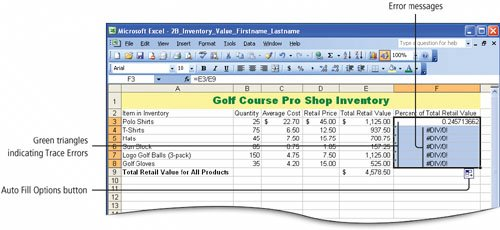Each cell displays an error message#DIV/0!; each cell also displays a Trace Error triangle in the upper left corner, and the Auto Fill Options button displays. 4. Click cell F4, and then point to the Trace Error button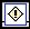to display its ScreenTip, as shown in Figure 2.29.   Figure 2.29.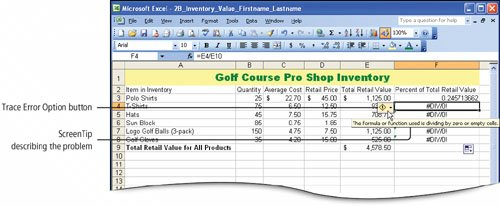The ScreenTip indicates The formula or function used is dividing by zero or empty cells. 5. Look at the Formula Bar to examine the formula. The formula is =E4/E10. The cell reference to E4 is correct, but the cell reference following the division operator (/) is E10, and E10 is an empty cell. 6. Click cell F5, and in the Formula Bar examine the formula =E5/E11.   Because the cell references are relative, Excel attempts to build the formulas by increasing the row number for each equation. In this particular calculation, however, the divisor must always be the value in cell E9the Total Retail Value for All Products. 7. Point to cell F3 and double-click to have the range finder display the cell's formula and place the insertion point within the cell. Alternatively, click in the Formula Bar to place the insertion point for editing the formula there. 8. Edit the formula so that it indicates =E3/\$E\$9 and then compare your screen with Figure 2.30.   Figure 2.30.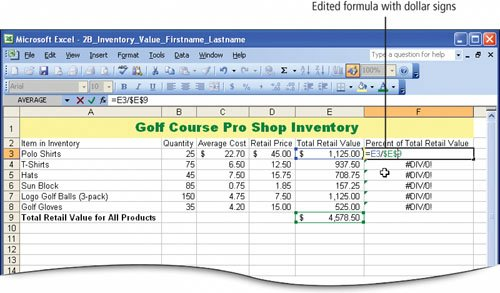To make a cell reference absolute, dollar signs are inserted into the cell reference. The use of the dollar sign to denote an absolute reference is not related in any way to whether or not the values you are working with are currency values. It is simply the symbol used by Excel to denote an absolute cell reference. 9. On the Formula Bar, click the Enter button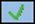so that F3 is still the active cell. Then, drag the fill handle to copy the formula down through cell F8. Compare your screen with Figure 2.31.     Figure 2.31.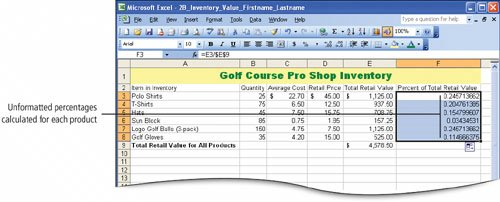10. Click in several of the copied cells and look at the formula in the Formula Bar. You can see that for each formula, the cell reference for the Total Retail Value of each product changed relative to its row, but the value used as the divisorTotal Retail Value for All Products in cell E9remained absolute. Thus, using either relative or absolute cell references, it is easy to duplicate formulas without typing them. 11. Saveyour workbook.

Another Way: To Make a Cell Reference Absolute

You can make a cell reference absolute while creating the formula. Click to select the cell to which you want to refer, press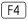, and then continue creating the formula. Excel will insert the dollar signs indicating an absolute reference for you.

[Page 726 (continued)]

### Objective 7 Format Percents, Move Formulas, and Wrap Text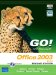GO! with Microsoft Office 2003 Brief (2nd Edition)
ISBN: 0131878646
EAN: 2147483647
Year: 2004
Pages: 448